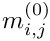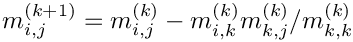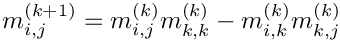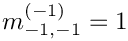GiNaC 1.8.7
GiNaC::determinant_algo Class Reference

Switch to control algorithm for determinant computation. More...

`#include <flags.h>`

## Public Types

enum  {
automatic , gauss , divfree , laplace ,
bareiss
}

## Detailed Description

Switch to control algorithm for determinant computation.

Definition at line 88 of file flags.h.

## ◆ anonymous enum

 anonymous enum
Enumerator
automatic

Let the system choose.

A heuristics is applied for automatic determination of a suitable algorithm.

gauss

Gauss elimination.

Ifare the entries of the original matrix, then the matrix is transformed into triangular form by applying the rulesThe determinant is then just the product of diagonal elements. Choose this algorithm only for purely numerical matrices.

divfree

Division-free elimination.

This is a modification of Gauss elimination where the division by the pivot element is not carried out. Ifare the entries of the original matrix, then the matrix is transformed into triangular form by applying the rulesThe determinant can later be computed by inspecting the diagonal elements only. This algorithm is only there for the purpose of cross-checks. It is never fast.

laplace

Laplace elimination.

This is plain recursive elimination along minors although multiple minors are avoided by the algorithm. Although the algorithm is exponential in complexity it is frequently the fastest one when the matrix is populated by complicated symbolic expressions.

bareiss

Bareiss fraction-free elimination.

This is a modification of Gauss elimination where the division by the pivot element is delayed until it can be carried out without computing GCDs. Ifare the entries of the original matrix, then the matrix is transformed into triangular form by applying the rules(We have setin order to avoid a case distinction in above formula.) It can be shown that nothing more than polynomial long division is needed for carrying out the division. The determinant can then be read of from the lower right entry. This algorithm is rarely fast for computing determinants.

Definition at line 90 of file flags.h.

The documentation for this class was generated from the following file:

This page is part of the GiNaC developer's reference. It was generated automatically by doxygen. For an introduction, see the tutorial.